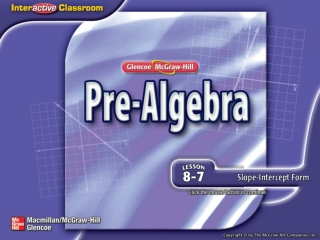DownloadDownload PresentationSplash Screen

# Splash Screen

Download Presentation## Splash Screen

- - - - - - - - - - - - - - - - - - - - - - - - - - - E N D - - - - - - - - - - - - - - - - - - - - - - - - - - -
##### Presentation Transcript

1. Splash Screen

2. Five-Minute Check (over Lesson 8–6) Then/Now New Vocabulary Key Concept: Slope-Intercept Form Example 1: Find the Slope and y-intercept Example 2: Find Slope and y-intercept Example 3: Graph an Equation Example 4: Analyze the Slope and y-intercept Lesson Menu

3. A.1 B. C. D. Find the slope of the line on the graph. 5-Minute Check 1

4. A. B. C. D. Find the slope of the line that passes through E(1, 4) and F(5, –2). 5-Minute Check 2

5. A.0 B. C.1 D.–1 Find the slope of the line that passes through G(0, –7) and H(2, –7). 5-Minute Check 3

6. A.0 B. C.1 D.–1 Find the slope of the line that passes through J(0, 0) and K(4, –4). 5-Minute Check 4

7. A. B. C. D. Find the slope of a line that decreases 4 units vertically for every 10-unit horizontal increase. 5-Minute Check 5

8. A.6 B.3 C. D. A hill rises at a vertical distance of 4 feet over a horizontal distance of 24 feet. What is the slope of the hill? 5-Minute Check 6

9. You have already graphed linear equations using ordered pairs. (Lesson 8–3) • Determine slopes and y-intercepts of lines. • Graph linear equations using the slope and y-intercept. Then/Now

10. slope-intercept form Vocabulary

11. Concept

12. State the slope and the y-intercept of the graph of Find the Slope and y-intercept Write the original equation. Answer: Example 1

13. State the slope and the y-intercept of the graph of y = 2x – 7. A.m = 2; b = –7 B.m = 2; b = 7 C.m = 7; b = 2 D.m = –7; b = 2 Example 1

14. Find Slope and y-intercept State the slope and the y-intercept of the graph of –4x + y = –10. –4x + y = –10 Write the original equation. –4x + y + 4x = –10 + 4x Add 4x to each side. y = –10 + 4x Simplify. Example 2

15. Find Slope and y-intercept y = 4x – 10 Write the equation in slope-intercept form. m = 4, b = –10 Answer: The slope of the graph is 4 and the y-intercept is –10. Example 2

16. State the slope and the y-intercept of the graph of –5x + y = 1. A.m = –5; b = 1 B.m = 5; b = 1 C.m = 1; b = 5 D.m = 1; b = –5 Example 2

17. Graph an Equation Graph y = –3x + 9 using the slope and y-intercept. Step 1 Find the slope and y-intercept. slope = –3 y-intercept = 9 Step 2 Graph the y-intercept point at (0, 9). Example 3

18. Graph an Equation Answer: Step 3 Use it to locate a second point on the line. Another point on the line is at (1, 6). Step 4 Draw a line through the two points and extend the line. Example 3

19. How would you graph y = 2x + 6? A. Graph a point at (6, 0). Move down two units and right one unit. Draw a line through the two points. B. Graph a point at (6, 0). Move left two units and up one unit. Draw a line through the two points. C. Graph a point at (0, 6). Move up two units and right one unit. Draw a line through the two points. D. Graph a point at (0, 6). Move left two units and up one unit. Draw a line through the two points. Example 3

20. Analyze the Slope and y-intercept A. BAKING A class spends \$75 on ingredients and then sells cookies for \$5 a dozen. The amount earned y can be represented by the equation y = 5x – 75, where x equals the number of dozens sold. Graph the equation. Step 1 First, find the slope and the y-intercept. slope = 5 y-intercept = –75 Example 4 A

21. Step 2 Plot the point at (0, –75). Since , go up 50 and right 10 and plot another point. Analyze the Slope and y-intercept Step 3 Connect these points and extend the line. Answer: Example 4 A

22. Analyze the Slope and y-intercept B. Describe what the y-intercept and the slope represent in the graph. Answer: The y-intercept –75 represents the cost of the ingredients. Slope 5 represents the dollars earned per dozen cookies. Example 4 B

23. A.B. C.D. A. T-SHIRTS A T-shirt company spends \$150 on materials to make T-shirts and then sells the shirts for \$12 each. The amount earned y can be represented by the equation y = 12x – 150, where x represents the number of shirts sold. Graph the equation. Example 4 CYP A

24. B. Describe what the y-intercept and the slope represent in the graph. A.y-intercept 150 represents number of shirts sold; slope 12 represents cost of materials. B.y-intercept –150 represents cost of materials; slope 12 represents dollars earned per shirt. C.y-intercept 150 represents dollars earned; slope 12 represents cost per shirt. D.y-intercept –150 represents cost of materials; slope 12 represents number of shirts sold. Example 4 CYP B

25. End of the Lesson